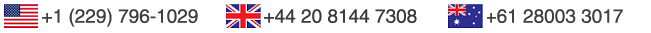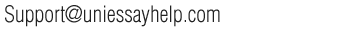Order now# Write a recursive version of this method.

Java Recursive Problems (4 problems):

Problem  I.    We define the Shaw strings as follows:

1. abb is a Shaw string.

2. bca is a Shaw string.

3. If S is a Shaw string, so is SaS.

4. If U and V are Shaw strings, so is UbV.

Here a, b, c are constants and S,U,V are variables. In these rules,

the same letter represents the same string. So, if S = abb,

rule 3 tells us that abbaabb is a Shaw string.

In rule 4, U and V represent Shaw strings, but they may be different.

Write the method

public static boolean isShaw(String in)

that returns true if in is a Shaw string and false otherwise.

Problem II.

public static  double getLargest(double [] a, int low, int high)

that returns the largest number in the array slice a[low:high].

If low > high, it throws an IllegalArgumentException. Otherwise, it checks if

the slice has 1 item. If so, it returns that value.

If the slice has 2 or more items, it divides the slice into 2 equal subslices,

computes the largestvalues of the 2 subslices and returns the largest of the 2 values.

Problem III. We define the Raju numbers as

Raju(0) = 1

Raju(1) =1

Raju(n) = Raju(n-1) + Raju(n-2) + 3 if n >= 2

public static long Raju(int n)

returns the n-th Raju number. If n < 0, the method throws an IllegalArgumentException.

Problem IV. Write the method

public static int binarySearch(double[] arr, int low, int high, double inq)

that search the array slice arr[low:high] for an occurrence

of inq. If inq occurs in arr, return an index i such that

arr[i] == inq. Otherwise, return -1.

Assume that arr is sorted in increasing order. Write a recursive version of this method.

The Output:

run:

Checking Hw

==============

We check isShaw

isShaw(“abbbabb”) is true

isShaw(“abbbaca”) is false

isShaw(“abbbabbaabbbabb”) is true

isShaw(“abbbbcabca”) is false

isShaw(“abbbbcaaabbaabb”) is false

isShaw(“abbbbcaabca”) is true

We test getLargest

The array is arr = { 6.9, -10.3, 6.7, 2.5, 16.4, -3.1}.

The largest of arr[0:6] is

We got an illegal argument exception.

The largest of arr[2:1] is

We got an illegal argument exception.

The largest of arr[2:2] is 6.7

The largest of arr[0:4] is 16.4

We test the Raju numbers.

We test the Raju numbers.

Raju(-1) is

We got an illegal argument exception.

Raju(2) is 5

Raju(4) is 17

Raju(10) is 353

We test binary search.

The input array is table = { 2, 4, 6, 8, 10, 12, 14 }.

2 was found in table[0:6] at index 0

3 was found in table[0:6] at index -1

4 was found in table[2:6] at index -1

12 was found in table[2:5] at index 5

This is all folks. I hope that your program worked.

BUILD SUCCESSFUL (total time: 2 seconds)

Place a similar order with us or any form of academic custom essays related subject and it will be delivered within its deadline. All assignments are written from scratch based on the instructions which you will provide to ensure it is original and not plagiarized. Kindly use the calculator below to get your order cost; Do not hesitate to contact our support staff if you need any clarifications.